# 2.3. COMPUTER ARITHMETICNo matter in which the system of numbers running and enter data in a digital computer, and what will be the electronic circuits to apply, all operations are carried out in a computer in binary number system the reasons mentioned above. Essentially there is no difference in the process of performing arithmetic operations in the binary system of numbers and the decade (or someone else), because all the rules that apply in the basic arithmetic operations in decade system of numbers apply to the binary number system.

# 2.3.1. Binary arithmeticArithmetic operation with binary numbers are simple, only need to be followed mathematical rules, such as:Binary account 1 + 1 = 10 should be understood as when the decade number system fills ten (baseline), for example 5 + 5 = 10, five over five equals zero, one on. Binary account 11 + 1 = 100 is analogous to the example in decade 45 + 55 = 100.

Example I

Converting decade number 15 and 5 in binary and basic mathematical operations with them:Analogously given below: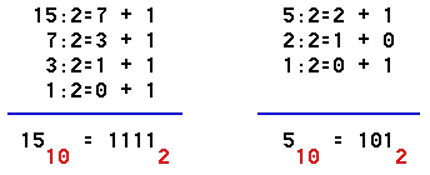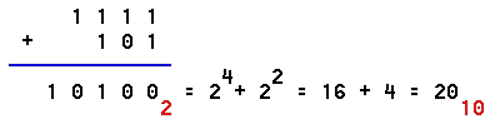b.) subtraction: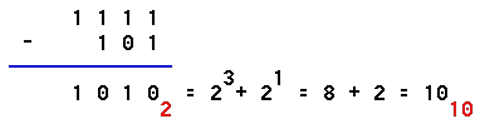c.) multiplication: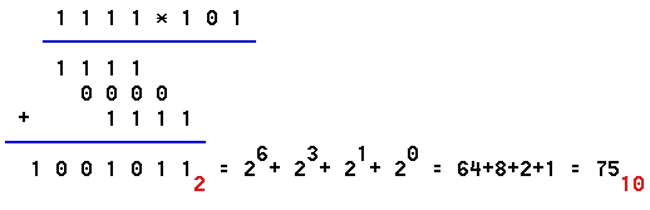d.) division: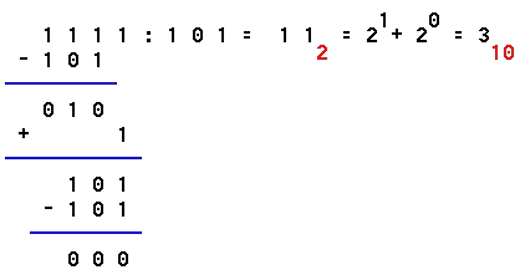Following the example of arithmetic operations in the binary numeral system in anything are not different from computer operations into decimal number system. Does it still make sense to explain other mathematical operations? No, especially because the binary number system used in the logic of computer components, with which essentially deal only with the designers of computer chips, very small and specialized type of experts. To them are required more thorough knowledge of this field. For users of computers it is only important to know that the mathematical operations in a digital computer system performs in the binary number system.

Computer multiplication and division comes down to the successive addition and subtraction, and a variety of methods can be reduced to the summation of subtraction, which greatly simplifies the arithmetic logic circuit of chips. Thus, the digital computer used in arithmetic operations primarily summing numbers. Of course, the process is longer, but it is of no importance to the user because of the fascinating speed with which the computer account.Citing of this page: Radic, Drago. " IT - Informatics Alphabet " Split-Croatia. {Date of access}. . Copyright © by Drago Radic. All rights reserved. | Disclaimer